# Predictive Modeling in R for Algorithmic Trading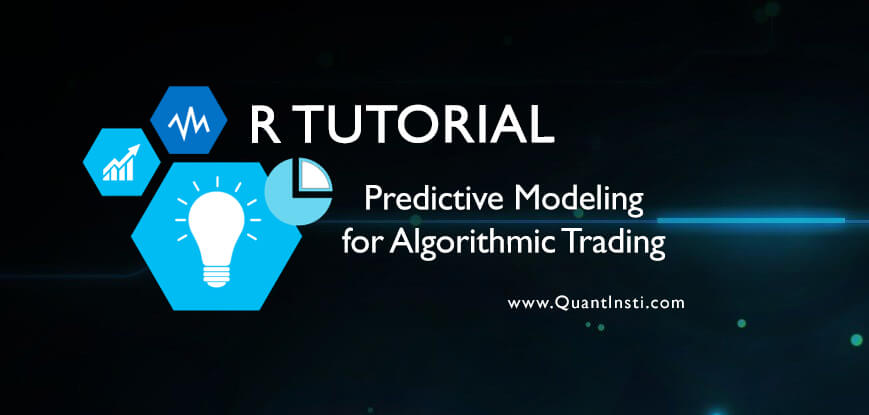### What is Predictive Modeling?

Predictive modeling is a process used in predictive analytics to create a statistical model of future behavior. Predictive analytics is the area of data mining concerned with forecasting probabilities and trends 

The predictive modeling in algorithmic trading is a modeling process wherein we predict the probability of an outcome using a set of predictor variables. In this post, we will be illustrating predictive modeling in R.

### Who should use it?

Predictive models can be built for different assets like stocks, futures, currencies, commodities etc. For example, we can build a model to predict the next day price change for a stock, or a model to predict the foreign currency exchange rates.

### How/Why should we use it?

The power of predictive modeling can be harnessed for making the right investment decisions, and in building profitable portfolios.

In this article, we will be detailing the step-by-step process for predictive modeling in R used for trading using different technical indicators. This model attempts to predict the next day price change (Up/Down) using these indicators and machine learning algorithms.

#### Step 1: Feature construction

Our process commences with the construction of a dataset that contains the features which will be used to make the predictions, and the output variable.

First, we build our dataset using raw data comprising of a 5-year price series for a stock and an index. This stock and index data consists of Date, Open, High, Low, Last and Volume. Using this data we compute our features based on various technical indicators (listed below).

 No Name of the Technical Indicator 1 Force Index(FI) 2 William %R 3 Relative Strength Index(RSI) 4 Rate Of Change(ROC) 5 Momentum (MOM) 6 Average True Range(ATR)

Note: Before you begin, make sure that you have the following packages installed and selected on your RStudio: Quantmode, PRoc, TTR, Caret, Corrplot, FSelector, rJava, kLar, randomforest, kernlab, rpart

##### R Code: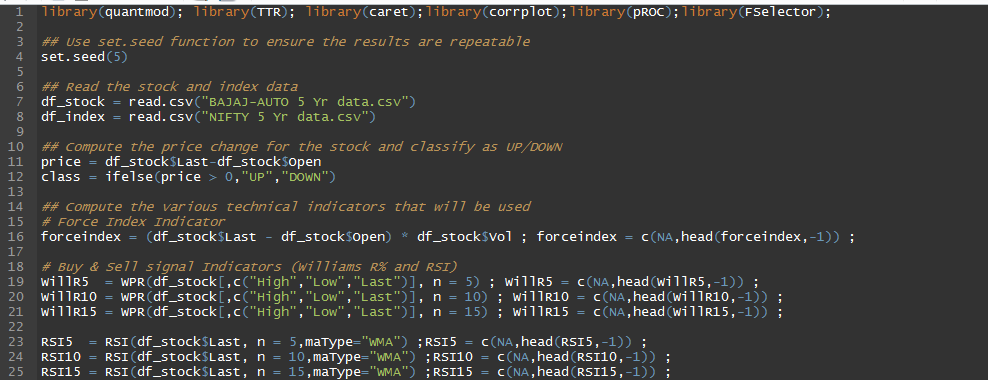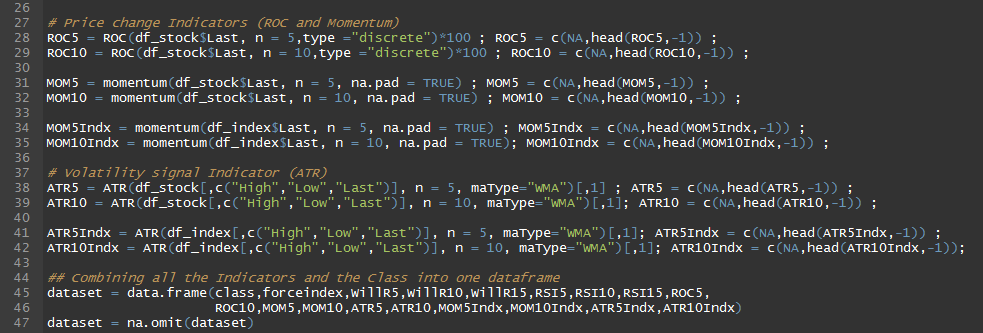The computed technical indicators along with the price change class (Up/Down) are combined to form a single dataset.

#### Step 2: Understanding the dataset using numbers and visuals

The most significant pre-requisite for predictive modeling is to have a good understanding of the dataset. The understanding helps in:

• Data transforms
• Choosing the right machine learning algorithms
• Explains the results obtained from the model
• Improves its accuracy

To attain this understanding of the dataset, you can use descriptive statistics like standard deviation, mean, skewness, along with graphical understanding of the data.

##### R Code: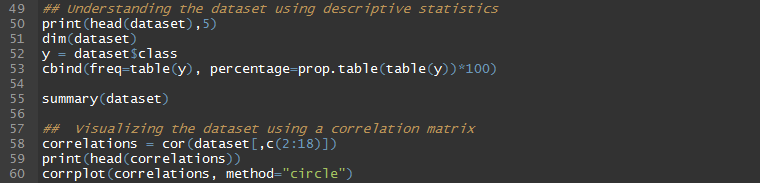Correlation Matrix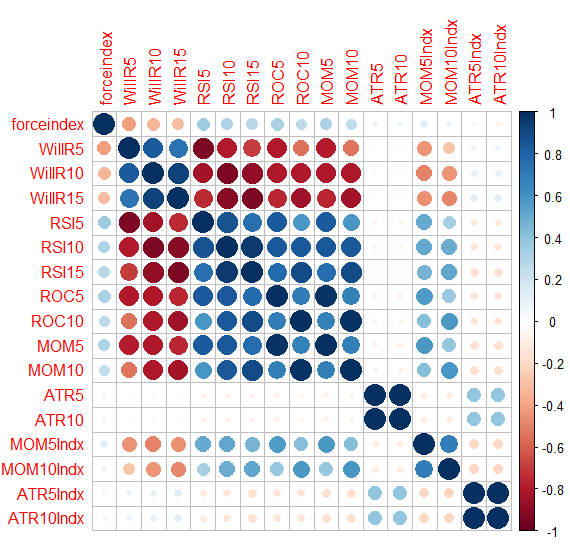#### Step 3: Feature selection

Feature selection is the process of selecting a subset of features that are most relevant for model construction which aid in creating an accurate predictive model. There are a wide range of feature selection algorithms, and these mainly fall in one of the three categories:

1. Filter method– selects features by assigning a score to them using some statistical measure.
2. Wrapper method– evaluates different subset of features, and determines the best subset.
3. Embedded method – This method figures out which of the features give the best accuracy while the model is being trained.

In our model, we will use filter method utilising the random.forest.importance function from the FSelector package. The random.forest.importance function rates the importance of each feature in the classification of the outcome, i.e. class variable. The function returns a data frame containing the name of each attribute and the importance value based on the mean decrease in accuracy.

##### R Code: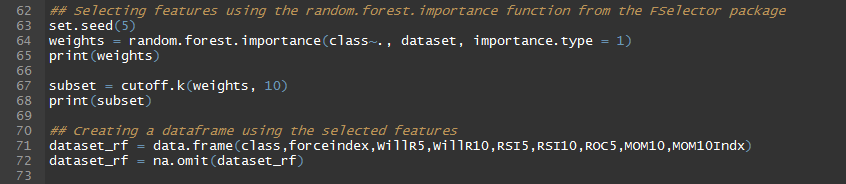##### Output: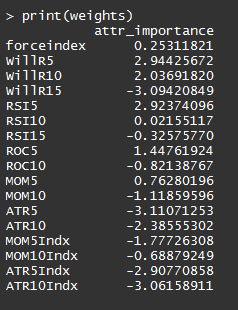Now, in order to choose the best features using the importance values returned by random.forest.importance, we use the cutoff.k function which provides k features with the highest importance values.

In our model, we have selected ten out of the seventeen features that were initially extracted from the price data series. Using these ten features we create a dataset that will be used on the machine learning algorithms.

#### Step 4: Set the Resampling method

In Predictive modeling we need data for two reasons:

• To train the model
• To test data to determine the accuracy of the predictions made by the model.

When we have a limited data we can use resampling methods which split data into training and testing parts. There are different resampling methods available in R such as data splitting, bootstrap method, k-fold cross validation, Repeated k-fold cross validation etc.

In our example, we are using k-fold cross validation method which splits the dataset into k-subsets. We keep each subset aside while the model trains on all the remaining subsets. This process repeats until it determines accuracy for each instance in the dataset. Finally, the function determines an overall accuracy estimate.

#### Step 5: Training different algorithms

There are hundreds of machine learning algorithms available in R, and determining which model to use can be confusing for beginners. Modelers are expected to try different algorithms based on the problem at hand and with more experience & practice you will be able to determine the right set.

Few kinds of problems:

• Classification
• Regression
• Clustering
• Rule Extraction

In our example we are dealing with a classification problem, hence we will explore some algorithms that are geared to solve such problems.  We will be using the following:

• k-Nearest Neighbors (KNN)
• Classification and Regression Trees (CART)
• Naive Bayes (NB)
• Support Vector Machine with Radial Basis Function (SVM) algorithms.

We won’t be detailing the working of these algorithms as the objective of the post is to detail the steps in the modeling process, and not the underlying working of these algorithms.

We use the train function from the caret package which fits different predictive models using a grid of tuning parameters. For example: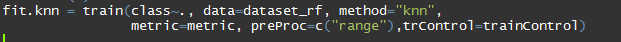In the above example, we are using the KNN algorithm which is specified via the method argument. class is the output variable, dataset_rf is the dataset that is used to train and test the model. The preProc argument defines the data transform method, while the trControl argument defines the computational nuances of the train function.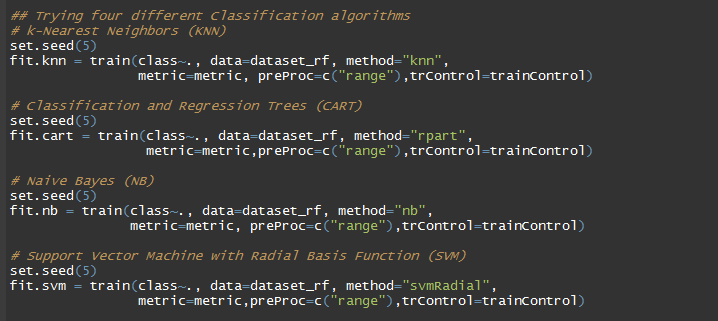#### Step 6: Evaluating the Models

The trained models are evaluated for their accuracy in predicting the outcome using different metrics like Accuracy, Kappa, Root Mean Squared Error (RMSE), R2etc.

We are using the “Accuracy” metric to evaluate our trained models. Accuracy is the percentage of correctly classified instances out of all instances in the test dataset. We have used the resamples function from the caret package which takes the trained objects, and produces a summary using the summary function.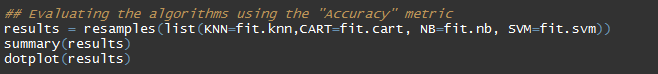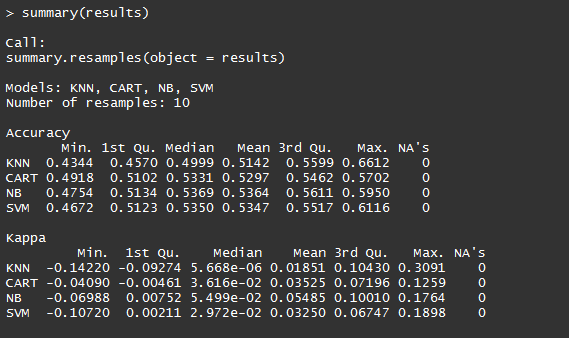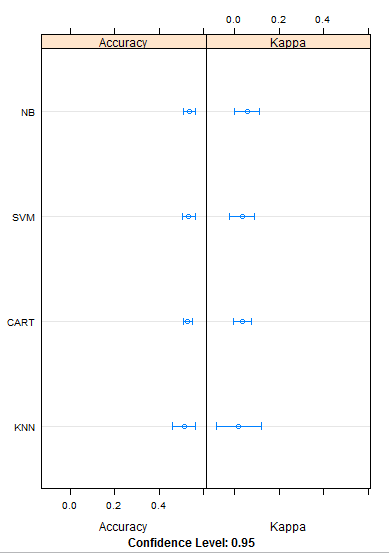#### Step 7: Tuning the shortlisted model

As we can observe from the accuracy metric, all the models have accuracy between 50-54%. Ideally we should try to tune models with highest accuracies. However, for the example’s sake, we will select the KNN algorithm and try to improve its accuracy by tuning the parameters.

##### Tuning the parameters

We will tune the KNN algorithm (parameter k) over values ranging from 1 to 10. The tuneGrid argument in the train function helps determine the accuracy over this range when evaluate the model.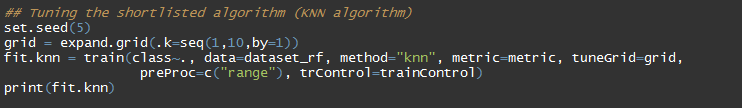##### Output:As can be seen from the tuning process, the accuracy of KNN algorithm has not increased and it is similar to the accuracy obtained earlier. You can try tuning other algorithms as well based on their respective tuning parameters, and select the algorithm with the best accuracy.

### Conclusion:

In the initial stages, modelers can try working with different technical indicators, create models based on other asset classes, and can try out different predictive modeling problems. There are some very good packages which make predictive modeling in R easy and with experience and practice, you will start building robust models to trade profitably in the markets.

### Next Step

Learn a simple to practice and implement Option trading strategy with an easy to follow example. You can also learn how to design a payoff chart for this strategy using Python Programming. Click here to access the strategy.